# Higher-Order Functions

Tips for navigating the slides:
• Press O or Escape for overview mode.
• Visit this link for a nice printable version
• Press the copy icon on the upper right of code blocks to copy the code

### Class outline:

• Iteration example
• Designing functions
• Generalization
• Higher-order functions
• Lambda expressions
• Conditional expressions

## Iteration example

### Virahaṅka-Fibonacci numbers

Discovered by Virahanka in India, 600-800 AD, later re-discovered in Western mathematics and commonly known as Fibonacci numbers.

0 1 1 2 3 5 8 13 21 34 …
+ =
+ =
+ =
+ =
+ =
+ =
+ =
+ =

### Virahanka's question

How many poetic meters exist for a total duration?

S = short syllable, L = long syllable

Duration Meters Total
1 S 1
2 SS, L 2
3 SSS, SL, LS 3
4 SSSS, SSL, SLS, LSS, LL 5
5 SSSSS, SSSL, SSLS, SLSS, SLL, LLS, LSL, LSSS 8

The So-called Fibonacci Numbers in Ancient and Medieval India

### Fibonacci's question

How many pairs of rabbits can be bred after N months?### Virahanka-Fibonacci number generation


VF 0   1   1   2   3   5   8   13   21   34   55 …
N  0   1   2   3   4   5   6   7    8    9    10 …


def vf_number(n):
"""Compute the nth Virahanka-Fibonacci number, for N >= 1.
>>> vf_number(2)
1
>>> vf_number(6)
8
"""
prev = 0  # First Fibonacci number
curr = 1  # Second Fibonacci number
k = 1
while k < n:
(prev, curr) = (curr, prev + curr)
k += 1
return curr


### Golden spiral

The Golden spiral can be approximated by Virahanka-Fibonacci numbers.### Go bears!

The Golden spiral is found everywhere in nature...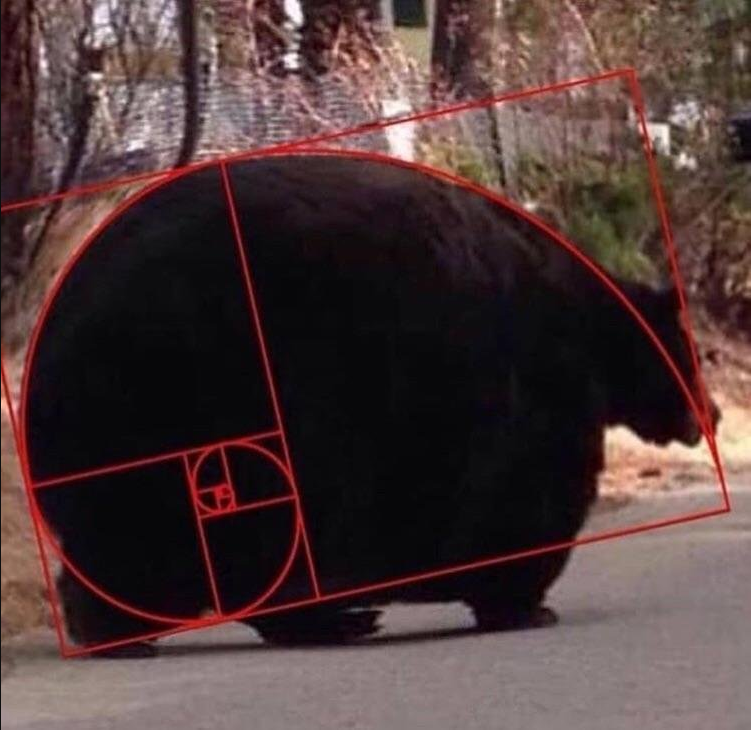## Designing Functions

### Describing Functions


def square(x):
"""Returns the square of X."""
return x * x

Aspect Example
A function's domain is the set of all inputs it might possibly take as arguments. x is a number
A function's range is the set of output values it might possibly return. square returns a non-negative real number
A pure function's behavior is the relationship it creates between input and output. square returns the square of x

### Designing a function

Give each function exactly one job, but make it apply to many related situations.


round(1.23)     # 1
round(1.23, 0)  # 1
round(1.23, 1)  # 1.2
round(1.23, 5)  # 1.23


Don't Repeat Yourself (DRY): Implement a process just once, execute it many times.

## Generalization

### Generalizing patterns with arguments

Geometric shapes have similar area formulas.

 Shape Area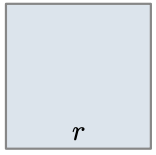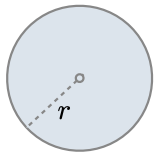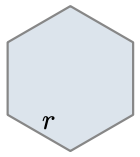$$\colorbox{#f8e9eb}{1} * r^2$$ $$\colorbox{#f8e9eb}{\pi} * r^2$$ $$\colorbox{#f8e9eb}{\dfrac{3\sqrt{3}}{2}} * r^2$$

### A non-generalized approach


from math import pi, sqrt

def area_square(r):
return r * r

def area_circle(r):
return r * r * pi

def area_hexagon(r):
return r * r * (3 * sqrt(3) / 2)


How can we generalize the common structure?

### Generalized area function


from math import pi, sqrt

def area(r, shape_constant):
"""Return the area of a shape from length measurement R."""
if r < 0:
return 0
return r * r * shape_constant

def area_square(r):
return area(r, 1)

def area_circle(r):
return area(r, pi)

def area_hexagon(r):
return area(r, 3 * sqrt(3) / 2)


## Higher-order functions

### What are higher-order functions?

A function that either:

• Takes another function as an argument
• Returns a function as its result

All other functions are considered first-order functions.

### Generalizing over computational processes

$$\sum\limits_{k=1}^5 \colorbox{#f8e9eb}{k} = 1 + 2 + 3 + 4 + 5 = 15$$ $$\sum\limits_{k=1}^5 \colorbox{#f8e9eb}{k^3} = 1^3 + 2^3 + 3^3 + 4^3 + 5^3 = 225$$ $$\sum\limits_{k=1}^5 \colorbox{#f8e9eb}{\dfrac{8}{(4k - 3)\cdot(4k - 1)}} = \dfrac{8}{3} + \dfrac{8}{35} + \dfrac{8}{99} + \dfrac{8}{195} + \dfrac{8}{323} = 3.04$$

The common structure among functions may be a computational process, not just a number.

### Functions as arguments


def cube(k):
return k ** 3

def summation(n, term):
"""Sum the first N terms of a sequence.
>>> summation(5, cube)
225
"""
total = 0
k = 1
while k <= n:
total = total + term(k)
k = k + 1


## Functions as return values

### Locally defined functions

Functions defined within other function bodies are bound to names in a local frame.


"""Return a function that takes one argument k
and returns k + n.
7
"""
return k + n


## Lambda expressions

### Lambda syntax

A lambda expression is a simple function definition that evaluates to a function.

The syntax:


lambda <parameters>: <expression>


A function that takes in parameters and returns the result of expression.

A lambda version of the square function:


square = lambda x: x * x


A function that takes in parameter x and returns the result of x * x.

### Lambda syntax tips

A lambda expression does not contain return statements or any statements at all.

Incorrect:


square = lambda x: return x * x

Correct:

square = lambda x: x * x


### Def statements vs. Lambda expressions


def square(x):
return x * x

vs

square = lambda x: x * x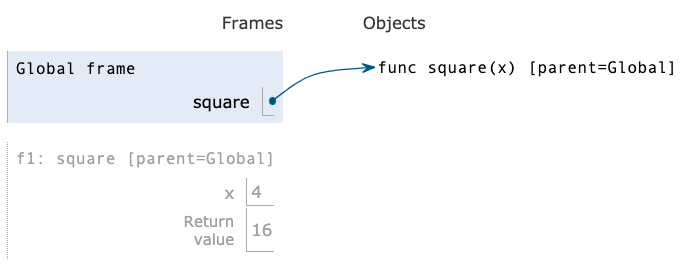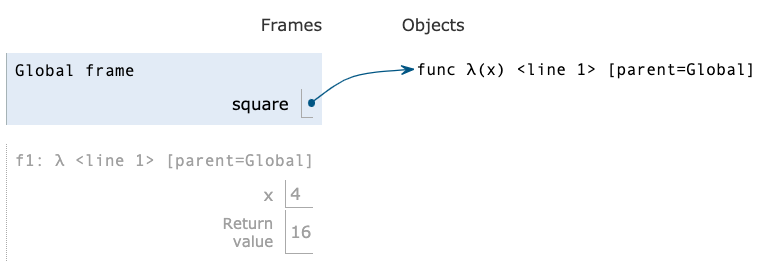Both create a function with the same domain, range, and behavior.
Both bind that function to the name square.
Only the def statement gives the function an intrinsic name, which shows up in environment diagrams but doesn't affect execution (unless the function is printed).

### Lambda as argument

It's convenient to use a lambda expression when you are passing in a simple function as an argument to another function.


def cube(k):
return k ** 3

summation(5, cube)


We can use a lambda:


summation(5, lambda k: k ** 3)


## Conditional expressions

### Conditional expressions

A conditional expression has the form:


<consequent> if <predicate> else <alternative>


Evaluation rule:

• Evaluate the <predicate> expression.
• If it's a true value, the value of the whole expression is the value of the <consequent>.
• Otherwise, the value of the whole expression is the value of the <alternative>.

### Lambdas with conditionals

This is invalid syntax:


lambda x: if x > 0: x else: 0


Conditional expressions to the rescue!


lambda x: x if x > 0 else 0